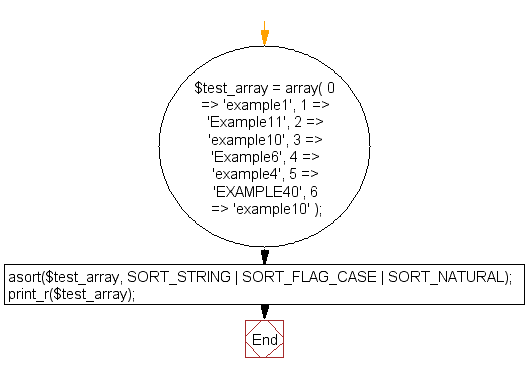﻿ PHP Array Exercise: Sort an associative array by values - w3resource# PHP Array Exercises : Sort an associative array by values

## PHP Array: Exercise-34 with Solution

Write a PHP program to sort an associative array (alphanumeric with case-sensitive data) by values.

Sample Solution:

PHP Code:

``````<?php
\$test_array = array(
0 => 'example1',
1 => 'Example11',
2 => 'example10',
3 => 'Example6',
4 => 'example4',
5 => 'EXAMPLE40',
6 => 'example10'
);
asort(\$test_array, SORT_STRING | SORT_FLAG_CASE | SORT_NATURAL);
print_r(\$test_array);
?>
```
```

Sample Output:

```Array
(
 => example1
 => example4
 => Example6
 => example10
 => example10
 => Example11
 => EXAMPLE40
)
```

Flowchart:PHP Code Editor:

What is the difficulty level of this exercise?

﻿

## PHP: Tips of the Day

PHP: Extract numbers from a string

```\$str = 'In My Cart : 11 12 items';
preg_match_all('!\d+!', \$str, \$matches);
print_r(\$matches);
```